CRR Tool
Capital Requirements Regulation (CRR)
Article 325r

# Article 325r - Delta risk sensitivities

Status
In force
Selected consolidated version from
30/09/2021
Search all articles and keywords within this legal act

Article 325r

Delta risk sensitivities

1.

Institutions shall calculate delta general interest rate risk (GIRR) sensitivities as follows:

(a)

the sensitivities to risk factors consisting of risk-free rates shall be calculated as follows: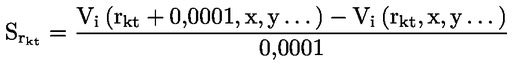where: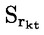= the sensitivities to risk factors consisting of risk-free rates;
 rkt = the rate of a risk-free curve k with maturity t;
 Vi (.) = the pricing function of instrument i; and
 x,y = risk factors other than rkt in the pricing function Vi;
(b)

the sensitivities to risk factors consisting of inflation risk and cross-currency basis shall be calculated as follows: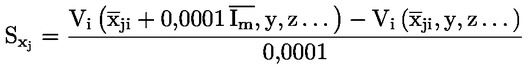where: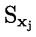= the sensitivities to risk factors consisting of inflation risk and cross-currency basis;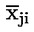= a vector of m components representing the implied inflation curve or the cross-currency basis curve for a given currency j with m being equal to the number of inflation or cross-currency related variables used in the pricing model of instrument i;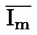= the unity matrix of dimension (1 × m);
 Vi (.) = the pricing function of the instrument i; and
 y, z = other variables in the pricing model.
2.

Institutions shall calculate the delta credit spread risk sensitivities for all securitisation and non-securitisation positions as follows: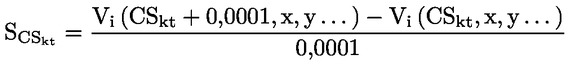where: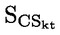= the delta credit spread risk sensitivities for all securitisation and non-securitisation positions;
 cskt = the value of the credit spread rate of an issuer j at maturity t;
 Vi (.) = the pricing function of instrument i; and
 x,y = risk factors other than cskt in the pricing function Vi.
3.

Institutions shall calculate delta equity risk sensitivities as follows:

(a)

the sensitivities to risk factors consisting of equity spot prices shall be calculated as follows: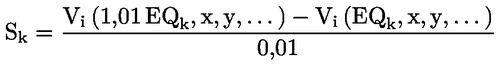where:

 sk = the sensitivities to risk factors consisting of equity spot prices;
 k = a specific equity security;
 EQk = the value of the spot price of that equity security;
 Vi (.) = the pricing function of instrument i; and
 x,y = risk factors other than EQk in the pricing function Vi;
(b)

the sensitivities to risk factors consisting of equity repo rates shall be calculated as follows: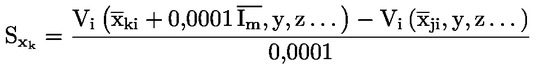where: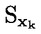= the sensitivities to risk factors consisting of equity repo rates;
 k = the index that denotes the equity;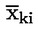= a vector of m components representing the repo term structure for a specific equity k with m being equal to the number of repo rates corresponding to different maturities used in the pricing model of instrument i;= the unity matrix of dimension (1 · m);
 Vi (.) = the pricing function of the instrument i; and
 y,z = risk factors other thanin the pricing function Vi.
4.

Institutions shall calculate the delta commodity risk sensitivities to each risk factor k as follows: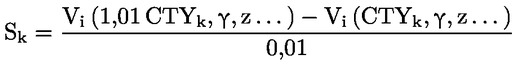where:

 sk = the delta commodity risk sensitivities;
 k = a given commodity risk factor;
 CTYk = the value of risk factor k;
 Vi (.) = the pricing function of instrument i; and
 y, z = risk factors other than CTYk in the pricing model of instrument i.
5.

Institutions shall calculate the delta foreign exchange risk sensitivities to each foreign exchange risk factor k as follows: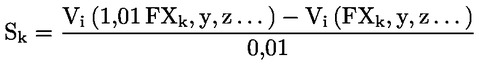where:

 sk = the delta foreign exchange risk sensitivities;
 k = a given foreign exchange risk factor;
 FXk = the value of the risk factor;
 Vi (.) = the pricing function of instrument i; and
 y, z = risk factors other than FXk in the pricing model of instrument i.